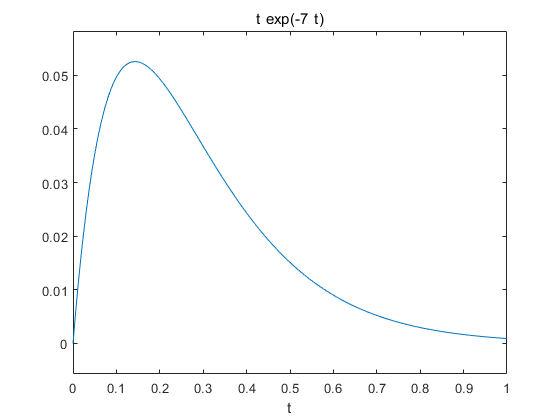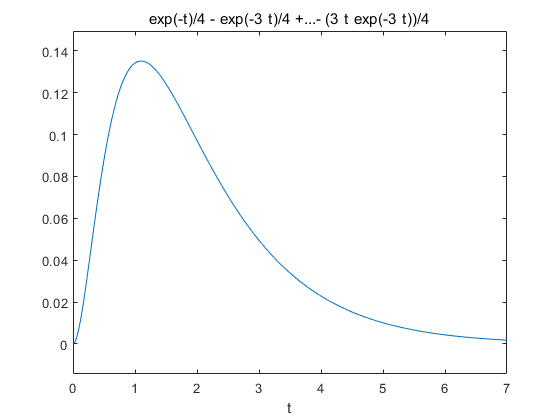# 为什么要进行拉普拉斯逆变换

• 有些函数中复频域中求解很困难，我们需要把它变换到时域中，或者想看它在时域中有什么特性
• 为了应付考试中的变态题目

# 函数名及使用步骤

• 使用syms 对变量进行定义
• 使用ilaplace()进行拉普拉斯逆变换

# 示例

• 简单的幂函数


octave:2> syms s;
octave:3> ilaplace(1/s^3)
ans = (sym)

2
t
──
2

• 简单的指数函数

 Octave的显示更=跟MATLAB不太一样

octave:4> syms w;
octave:5> ilaplace(2/(w+s))
ans = (sym)

-t⋅re(w) - ⅈ⋅t⋅im(w)
2⋅ℯ

• 三角函数

octave:7> ilaplace(s/(s^2+4))
ans = (sym) cos(2⋅t)


# 复杂函数的拉普拉斯逆变换

现实中有很多函数通过手算有很大的计算量，或者根本没有原函数，这个时候我们就只能通过MATLAB来求解

• 一个例子：octave:8> F = (5-3*s)/(2+5*s)
F = (sym)

5 - 3⋅s
───────
5⋅s + 2

octave:9> f = ilaplace(F)
f = (sym)

-2⋅t
─────
⎛   s               ⎞      5
- 3⋅InverseLaplaceTransform⎜───────, s, t, None⎟ + ℯ
⎝5⋅s + 2            ⎠



ilaplace(F)
ans = -3/5*dirac(t)+31/25*exp(-2/5*t)


###### 其中dirac(t)称为狄拉克函数，在自动控制和信号与系统中也叫单位脉冲函数
• 一个更复杂的例子：octave:11> F = (-s^2-9*s+4)/(s^2+s+2)
F = (sym)

2
- s  - 9⋅s + 4
──────────────
2
s  + s + 2

octave:12> f = ilaplace(F)
Waiting....!!! OUT OF TIME !!!


# 配合ezplot()函数绘制逆变换后的图像

ezplot能够进行符号计算，不需要自变量的数据就能根据函数特性绘制图像，但通常要给自变量指定区间


## 例1• 求解原函数

 syms s;
F = 1/(s+7).^2;
f = ilaplace(F);


f =

t*exp(-7*t)

• 绘制某个区间的图像

 ezplot(f)

• 图像## 例2• 代码

 syms s;
F = (2*s+3)/(((s+1).^2)*((s+3).^2));
f = ilaplace(F)
ezplot(f,[0 1])

f =

exp(-t)/4 - exp(-3*t)/4 + (t*exp(-t))/4 - (3*t*exp(-3*t))/4

• 图像06-14
10-07300603-27
03-19672
03-1642
09-075067
06-291967
05-08
08-26
01-263986
05-067万+
06-247万+
11-232096点击重新获取扫码支付余额充值